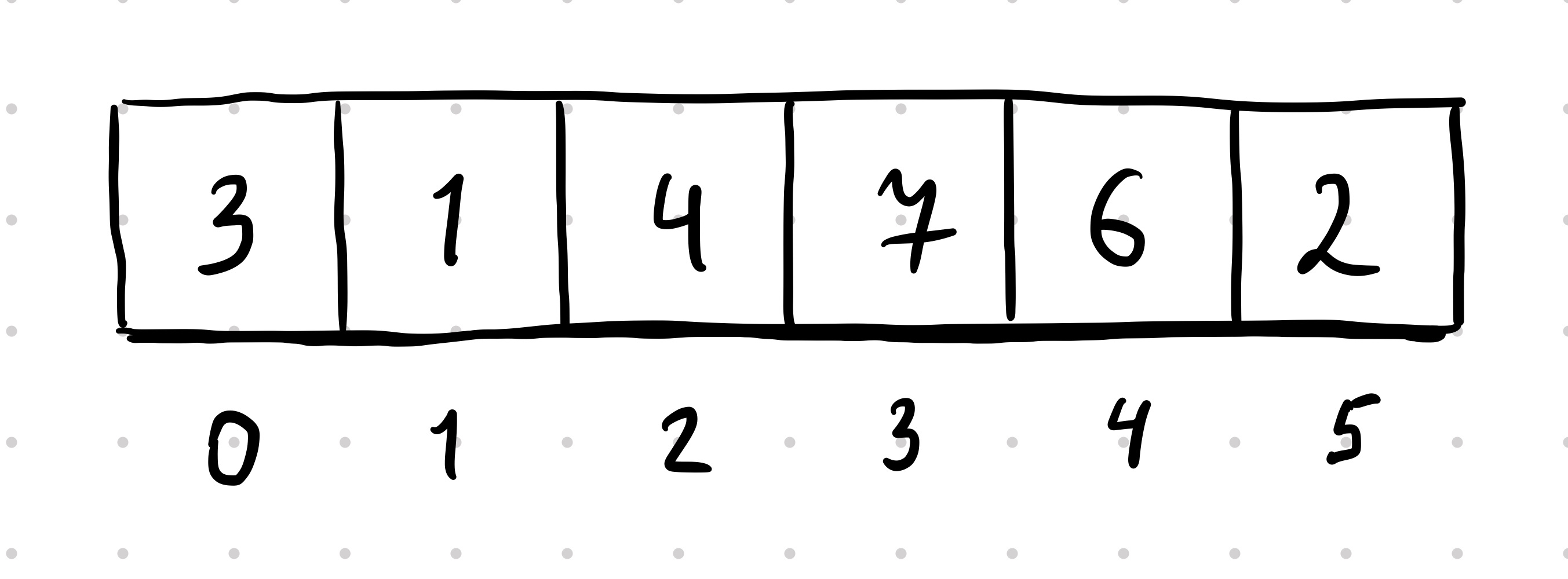###### Range Sum Query - Immutable ❄️

Given an integer array `nums`, handle multiple queries of the following type:

• Calculate the sum of the elements of `nums` between indices `left` and `right` inclusive where `left <= right`.

Examples for array `[-2, 0, 3, -5, 2, -1]`:

• `Query(0, 2) => 1`
• `Query(2, 5) => -1`
• `Query(0, 5) => -3`

Problem at Leetcode

##### Hi 👋
I can help you to build intuition for understanding how to implement an immutable range sum querier. You're queried to return sum range of elements from index 1 to index 3 inclusive. What's the answer?